# SSAT Middle Level Math : How to multiply fractions

## Example Questions

### Example Question #11 : How To Multiply Fractions

Raiseto the fourth power.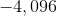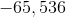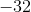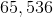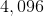Explanation:

To raise a negative number to an even-numbered power, raise its absolute value to that power: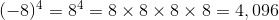### Example Question #12 : How To Multiply Fractions

Evaluate: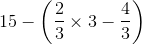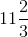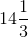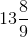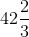Explanation:

By the order of operations, carry out the operations in parentheses first; since there is a multiplication and a subtraction present, carry them out in that order. Finally, carry out the remaining subtraction: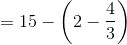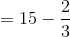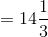### Example Question #4 : Multiplicative Inverse Property

Which of the following statements demonstrates the inverse property of multiplication?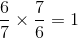None of the examples in the other responses demonstrates the inverse property of multiplication.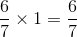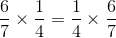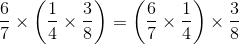Explanation:

The inverse property of multiplication states that for every real number, a number exists, called the multiplicative inverse, such that the number and its inverse have product 1. Of the statements given, onlydemonstrates this property.

### Example Question #13 : How To Multiply Fractions

Raise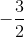to the fourth power.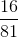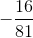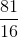Explanation:

To raise a negative number to an even-numbered power, raise its absolute value to that power. Also, to raise a fraction to a power, raise its numerator and its denominator to that power. Combine these ideas as follows: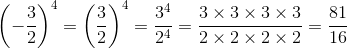### Example Question #14 : How To Multiply Fractions

Raise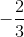to the fifth power.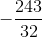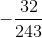cannot be raised to the fifth power.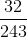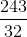Explanation:

To raise a negative number to an odd-numbered power, raise its absolute value to that power, then make the sign negative. Also, to raise a fraction to a power, raise its numerator and its denominator to that power. Combine these ideas as follows: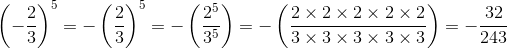### Example Question #15 : How To Multiply Fractions

Raiseto the fifth power.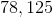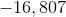cannot be raised to the fifth power.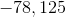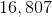Explanation:

To raise a negative number to an odd-numbered power, raise its absolute value to that power, then make the sign negative: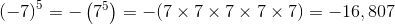### Example Question #16 : How To Multiply Fractions

One euro is worth approximately $1.27. For how much American money can a French tourist expect to exchange 800 euros? Possible Answers: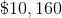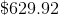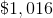The correct answer is not given among the other choices.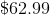Correct answer:Explanation: One Euro is equivalent to$1.27, so multiply the number of euros - 800 - by this conversion factor.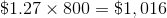### Example Question #17 : How To Multiply Fractions

Evaluate: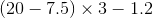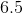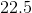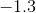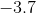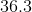Explanation:

By the order of operations, carry out the operation in parentheses, which is the leftmost subtraction, then the multiplication, then the rightmost subtraction: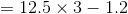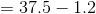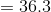### Example Question #18 : How To Multiply Fractions

Evaluate: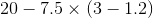Explanation:

By the order of operations, carry out the operation in parentheses, which is the rightmost subtraction, then the multiplication, then the leftmost subtraction: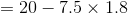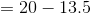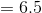### Example Question #3 : Multiplicative Identity Property

Which of the following statements demonstrates the identity property of multiplication?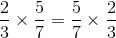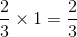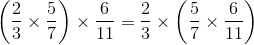None of the examples in the other responses demonstrates the identity property of multiplication.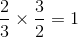Explanation:

The identity property of multiplication states that there is a number 1, called the multiplicative identity, that can be multiplied by any number to obtain that number. Of the four statements,demonstrates this property.

### All SSAT Middle Level Math Resources